Next: 6.4 BioFET Examples Up: 6. Generalization of the Previous: 6.2.6 Buffers and Ionic

# 6.3 Analytical Comparison between the Poisson-Boltzmann, the Extended Poisson-Boltzmann, and the Debye-Hückel Model

For better comparison between the Poisson-Boltzmann, the extended Poisson-Boltzmann, and the Debye-Hückel model we study their one-dimensional analytical solutions without any charges from macromolecules or due to the site-binding effect at the oxide surface. The surface potential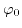will be chosen in a way that all models exhibit the same charge at the surface and that the potential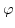and the electric field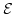vanish in the limit of infinite distance away from the surface. In the first step all equations are transformed to dimensionless units.

Reformulating the Laplace term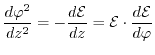(6.14)

and transforming the equations with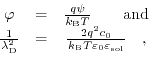(6.15)

leads to the following differential equations: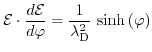(6.16)

for the Poisson-Boltzmann model,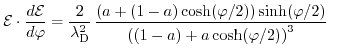(6.17)

for the extended Poisson-Boltzmann model and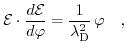(6.18)

for the Debye-Hückel model.

Assuming vanishing potentialand vanishing electric fieldfor large distances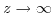, integrating these equations twice results in the following solutions: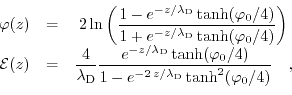(6.19)

for the Poisson-Boltzmann model ,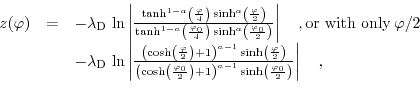(6.20)

or viaas a function of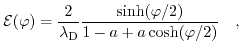(6.21)

for the extended Poisson-Boltzmann model , and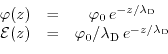(6.22)

for the Debye-Hückel model .

Unfortunately the analytical expression (6.27) is not as handy as the expressions (6.30) for the Debye-Hückel model and (6.25) for the Poisson-Boltzmann model. As can be seen in (6.27), only for the position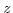as a function of the potentialit is possible to write down a compact analytical expression, while for the inverse function one has to use numerical approaches. However, in the limit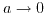the solution for the Poisson-Boltzmann model is recovered .

In the next step we assume an equivalent surface charge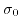for all three models, in order to accomplish a better comparison between them. This is realized by choosing an arbitrary charge at the surface and applying Gauß's law. This way, a surface potentialrelated to the same surface charge can be found.

The corresponding surface potentials are: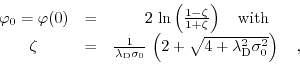(6.23)

for the Poisson-Boltzmann model ,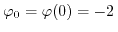arccosh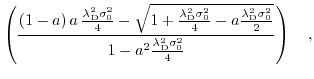(6.24)

for the extended Poisson-Boltzmann model , and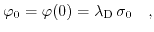(6.25)

the Debye-Hückel model, respectively.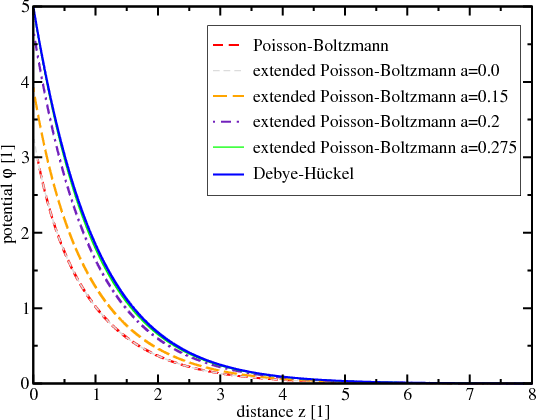Fig. 6.5 shows a comparison between the Poisson-Boltzmann, the extended Poisson-Boltzmann, and the Debye-Hückel model. As already mentioned before, one can see that forthe extended Poisson-Boltzmann model and the Poisson-Boltzmann model coincide. Increasing the closest possible approach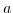between two ions, leads to a reduction in screening and thus higher surface potential. Furthermore, for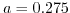the extended Poisson-Boltzmann model equations quite well with the Debye-Hückel model. This shows that the extended Poisson-Boltzmann model is able to cover a wider range of screening behavior than the Poisson-Boltzmann and the Debye-Hückel model.Next: 6.4 BioFET Examples Up: 6. Generalization of the Previous: 6.2.6 Buffers and Ionic

T. Windbacher: Engineering Gate Stacks for Field-Effect Transistors